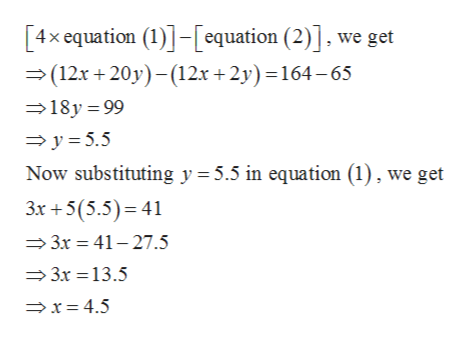# One month Tony rented 3 movies and 5 video games for a total of \$41. The next month he rented 12 movies and 2 video games for a total of \$65. Find the rental cost for each movie and each video game.

Question
30 views

One month Tony rented 3 movies and 5 video games for a total of \$41. The next month he rented 12 movies and 2 video games for a total of \$65. Find the rental cost for each movie and each video game.

check_circle

Step 1

Let \$x and \$y be the cost of each movie and video game respectively.

Step 2

Therefore, according to given data we get

Step 3

Solving the above two equa...help_outlineImage Transcriptionclose[4x equation (1)]-[equation (2)], we get (12x +20y)-12x +2y) =164-65 18y 99 y 5.5 Now substituting y 5.5 in equation (1), we get 3x 5(5.5) 41 3x 41-27.5 3x 13.5 x= 4.5 fullscreen

### Want to see the full answer?

See Solution

#### Want to see this answer and more?

Solutions are written by subject experts who are available 24/7. Questions are typically answered within 1 hour.*

See Solution
*Response times may vary by subject and question.
Tagged in

### Equations and In-equations